GeeksforGeeks App
Open AppBrowser
Continue

# CBSE Class 10 Term 1 Maths Answer Key 2021 Basic Paper

The CBSE Class 10 Mathematics Term 1 Exam for session 2021-22 was conducted by the Central Board of Secondary Education (CBSE) on 4th December 2021 Saturday for both Basic and Standard Mathematics Level. This year, more than 21 lakh students appeared for CBSE Class 10 Term 1 exam 2021. The Maths exam is the most important and tough exam for CBSE Class 10th students. Therefore, considering that CBSE has divided the Question paper into two Levels: Basic and Standard.

In 2019, the CBSE introduced two-level math (Standard and Basic) in Class 10 for the first time.

• Standard Maths is for those who wants to opt Mathematics as a subject in Classes 11 and 12.
• Basic Maths is for those who do not want to pursue the subject further.

This new approach was designed to make it easier for students who do not want to continue Mathematics in higher grades.

According to the guidelines, “Students who do not want to continue studying Mathematics in Grades 11 and 12 must pass the Mathematics Basic paper in Grade 10. If a student then changed their mind and wanted to study Math in Class 11, they were required to take the Class 10 Standard Mathematics paper in the compartment examinations.”

The CBSE Class 10 Maths Term 1 Paper had MCQ-type questions designed in different formats like questions based on assertion-reason, case study, and competency-based questions. The paper was of 40 marks with 40 questions to be attempted out of the given 50 questions. The question paper was divided into three sections as follows:

Teachers and experts who have reviewed the CBSE Class 10 Maths Standard and Basic examinations see it as a Balanced Paper. Only approximately 10-15% of the questions were difficult yet manageable, according to them. Conceptual questions were included in both standard and basic papers. The paper may have been easily solved by students who had a solid understanding of the fundamental concepts.

Hence, the question paper was long and relatively tough for the majority of students.

Here, the Solved Questions Paper (along with the Answers and the explanation in-depth for each question) of the Basic Maths CBSE Class 10th Term 1 Exam is provided as:

### Term- I

Time allowed- 90 minutes

Maximum Marks- 40

General Instructions

1. This question paper contains 50 questions out of which 40 questions are to be attempted. All questions carry equal marks.
2. The question paper consists of three Sections – Section A, B, and C.
3. Section – A contains 20 questions. Attempt any 16 questions from Q. No. 01 to 20.
4. Section – B also contains 20 questions. Attempt any 16 questions from Q. No. 21 to 40.
5. Section – C contains two Case Studies containing 5 questions in each case. Attempt any 4 questions from Q. No. 41 to 45 and another 4 from Q. No. 46 to 50.
6. There is only one correct option for every Multiple Choice Question (MCQ). Marks will not be awarded for answering more than one option.
7. There is no negative marking.

### SECTION – A

Question Number 1 to 20 are of 1 mark each. Any 16 Questions from Question 1 to 20 are needed to be attempted.

Question 1: HCF of 92 and 152 is

(a) 4

(b) 19

(c) 23

(d) 57

Question 2: In ΔABC, DE||BC, AD = 4 cm, DB = 6 cm and AE = 5 cm. The length of EC is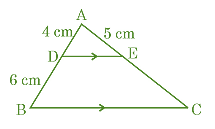(a) 7 cm

(b) 6.5 cm

(c) 7.5 cm

(d) 8 cm

Question 3: The value of k, for which the pair of linear equations x + y – 4 = 0, 2x + ky – 3 = 0 have no solution, is

(a) 0

(b) 2

(c) 6

(d) 8

Question 4: The value of (tan245° – cos260°) is

(a) 1/2

(b) 1/4

(c) 3/2

(d) 3/4

Question 5: A point (x, 1) is equidistant from (0, 0) and (2, 0). The value of x is

(a) 1

(b) 0

(c) 2

(d) 1/2

Question 6: Two coins are tossed together. The probability of getting exactly one head is

(a) 1/4

(b) 1/2

(c) 3/4

(d) 1

Question 7: A circular arc of length 22 cm subtends an angle θ at the centre of the circle of radius 21 cm. The value of θ is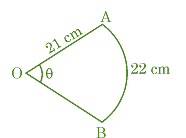(a) 90°

(b) 50°

(c) 60°

(d) 30°

Question 8: A quadratic polynomial having sum and product of its zeroes as 5 and 0 respectively, is

(a) x2 + 5x

(b) 2x(x – 5)

(c) 5x2 – 1

(d) x2 – 5x + 5

Question 9: If P(E) = 0.65, then the value of P(not E) is

(a) 1.65

(b) 0.25

(c) 0.65

(d) 0.35

Question 10: It is given that ΔDEF ~ ΔPQR. EF : QR = 3 : 2, then value of ar(DEF) : ar(PQR) is

(a) 4 : 9

(b) 4 : 3

(c) 9 : 2

(d) 9 : 4

Question 11: Zeroes of a quadratic polynomial x2 – 5x + 6 are

(a) –5, 1

(b) 5, 1

(c) 2, 3

(d) –2, –3

Question 12: 57/300 is a

(a) non-terminating and non-repeating decimal expansion.

(b) terminating decimal expansion after 2 places of decimals.

(c) terminating decimal expansion after 3 places of decimals.

(d) non-terminating but repeated decimal expansion.

Question 13: Perimeter of a rectangle whose length(l) is 4 cm more than twice its breadth(b) is 14 cm. The pair of linear equations representing the above information is

(a) l + 4 = 2b, 2(l + b) = 14

(b) l – b = 4, 2(l + b) = 14

(c) l = 2b + 4, l + b = 14

(d) l = 2b + 4, 2(l + b) = 14

Question 14:can also be written as

(a) 5.213213213…

(b) 5.2131313…

(c) 5.213

(d) 5213/1000

Question 15: The ratio in which the point (4, 0) divides the line segment joining the points (4, 6) and (4, –8) is

(a) 1 : 2

(b) 3 : 4

(c) 4 : 3

(d) 1 : 1

Question 16: Which of the following is not defined?

(a) sec 0°

(b) cosec 90°

(c) tan 90°

(d) cot 90°

Question 17: In the given figure, a circle is touching a semi-circle at C and its diameter AB at O. If AB = 28 cm, what is the radius of the inner circle?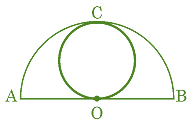(a) 14 cm

(b) 28 cm

(c) 7 cm

(d) 7/2 cm

Question 18: The vertices of a triangle OAB are O(0, 0), A(4, 0) and B(0, 6). The median AD is drawn on OB. The length AD is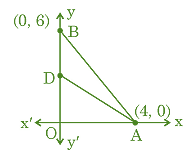(a) 52 units

(b) 5 units

(c) 25 units

(d) 10 units

Question 19: In a right-angled triangle PQR, ∠Q = 90°. If ∠P = 45°, then value of tan P – cos2R is

(a) 0

(b) 1

(c) 1/2

(d) 3/2

Question 20: If tan θ = 2/3, then the value of sec θ is

(a) √13/3

(b) √5/3

(c) √{13/3}

(d) 3/√13

### SECTION – B

Question numbers 21 to 40 are of 1 mark each. Any 16 Questions from Question 21 to 40 are needed to be Attempted:

Question 21: The perimeter of the sector of a circle of radius 14 cm and central angle of 45° is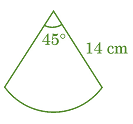(a) 11 cm

(b) 22 cm

(c) 28 cm

(d) 39 cm

Question 22: A bag contains 16 red balls, 8 green balls, and 6 blue balls. One ball is drawn at random. The probability that it is a blue ball is

(a) 1/6

(b) 1/5

(c) 1/30

(d) 5/6

Question 23: If sin θ – cos θ = 0, then the value of θ is

(a) 30°

(b) 45°

(c) 90°

(d) 0°

Question 24: The probability of happening of an event is 0.02. The probability of not happening of the event is

(a) 0.02

(b) 0.80

(c) 0.98

(d) 49/100

Question 25: Two concentric circles are centred at O. The area of the shaded region if outer and inner radii are 14 cm and 7 cm respectively, is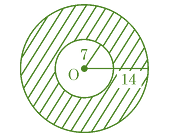(a) 462 cm2

(b) 154 cm2

(c) 231 cm2

(d) 308 cm2

Question 26: 1/(1 + sin θ) + 1/(1 – sin θ) can be simplified to get

(a) 2 cos2 θ

(b) 1/2 sec2 θ

(c) 2/sin2 θ

(d) 2 sec2 θ

Question 27: The origin divides the line segment AB joining the points A(1, –3) and B(–3, 9) in the ratio:

(a) 3 : 1

(b) 1 : 3

(c) 2 : 3

(d) 1 : 1

Question 28: The perpendicular bisector of a line segment A(–8, 0) and B(8, 0) passes through a point (0, k). The value of k is

(a) 0 only

(b) 0 or 8 only

(c) any real number

(d) any non-zero real number

Question 29: Which of the following is a correct statement?

(a) Two congruent figures are always similar.

(b) Two similar figures are always congruent.

(c) All rectangles are similar.

(d) The polygons having the same number of sides are similar.

Question 30: The solution of the pair of linear equations x = –5 and y = 6 is

(a) (–5, 6)

(b) (–5, 0)

(c) (0, 6)

(d) (0, 0)

Question 31: A circle of radius 3 units is centred at (0, 0). Which of the following points lie outside the circle?

(a) (–1, –1)

(b) (0, 3)

(c) (1, 2)

(d) (3, 1)

Question 32: The value of k for which the pair of linear equations 3x + 5y = 8 and kx + 15y = 24 has infinitely many solutions, is

(a) 3

(b) 9

(c) 5

(d) 15

Question 33: HCF of two consecutive even numbers is

(a) 0

(b) 1

(c) 2

(d) 4

Question 34: The zeroes of quadratic polynomial x2 + 99x + 127 are

(a) both negative

(b) both positive

(c) one positive and one negative

(d) reciprocal of each other

Question 35: The mid-point of line segment joining the points (–3, 9) and (–6, –4) is

(a) ( –3/2, –13/2 )

(b) ( 9/2, –5/2 )

(c) ( -9/2, 5/2 )

(d) ( 9/2, 5/2 )

Question 36: The decimal expansion of 13/(2 × 52 × 7) is

(a) terminating after 1 decimal place.

(b) non-terminating and non-repeating.

(c) terminating after 2 decimal places.

(d) non-terminating but repeating.

Question 37: In ΔABC, DE||BC, AD = 2 cm, DB = 3 cm, DE : BC is equal to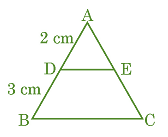(a) 2 : 3

(b) 2 : 5

(c) 1 : 2

(d) 3 : 5

Question 38: The (HCF × LCM) for the numbers 50 and 20 is

(a) 1000

(b) 50

(c) 100

(d) 500

Question 39: For which natural number n, 6n ends with digit zero?

(a) 6

(b) 5

(c) 0

(d) None

Question 40: (1 + tan2A) (1 + sin A) (1 – sin A) is equal to

(a) cos2A/sec2

(b) 1

(c) 0

(d) 2

### SECTION – C

Question numbers 41 to 45 and 46 to 50 are based on Case Study-I and Case Study-II. Here Any 4 Questions from each Case Study are needed to be attempted

Case Study – I

Sukriti throws a ball upwards, from a rooftop that is 8 m high from ground level. The ball reaches some maximum height and then returns and hits the ground. It height of the ball at time t (in sec) is represented by h(m), then the equation of its path is given as h = –t2 + 2t + 8 Based on the above information, answer the following: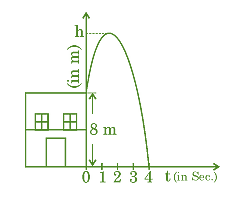tQuestion 41: The maximum height achieved by the ball is

(a) 7 m

(b) 8 m

(c) 9 m

(d) 10 m

Question 42: The polynomial represented by the above graph is

(a) linear polynomial

(c) constant polynomial

(d) cubic polynomial

Question 43: Time taken by the ball to reach maximum height is

(a) 2 sec.

(b) 4 sec.

(c) 1 sec.

(d) 2 min.

Question 44: Number of zeroes of the polynomial whose graph is given, is

(a) 1

(b) 2

(c) 0

(d) 3

Question 45: Zeroes of the polynomial are

(a) 4

(b) –2, 4

(c) 2, 4

(d) 0, 4

Case Study – II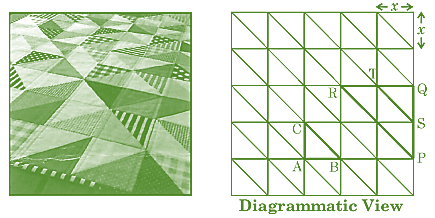Quilts are available in various colours and designs. The geometric design includes shapes like squares, triangles, rectangles, hexagons, etc. One such design is shown above. Two triangles are highlighted, ΔABC and ΔPQR. Based on the above information, answer the following questions:

Question 46: Which of the following criteria is not suitable for ΔABC to be similar to ΔQRP?

(a) SAS

(b) AAA

(c) SSS

(d) RHS

Question 47: If each square is of length x unit, then length BC is equal to

(a) x√2 unit

(b) 2x unit

(c) 2√x unit

(d) x√x unit

Question 48: Ratio BC : PR is equal to

(a) 2 : 1

(b) 1 : 4

(c) 1 : 2

(d) 4 : 1

Question 49: ar(PQR) : ar(ABC) is equal to

(a) 2 : 1

(b) 1 : 4

(c) 4 : 1

(d) 1 : 8

Question 50: Which of the following is not true?

(a) ΔTQS ~ ΔPQR

(b) ΔCBA ~ ΔSTQ

(c) ΔBAC ~ ΔPQR

(d) ΔPQR ~ ΔABC